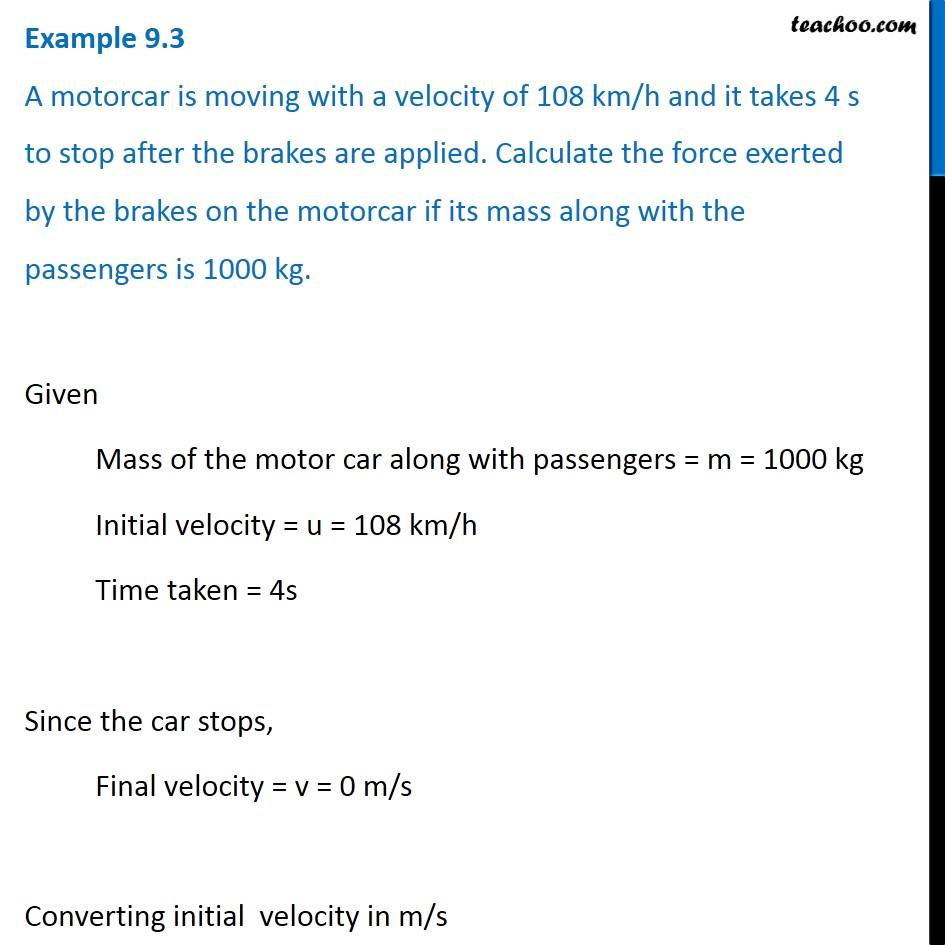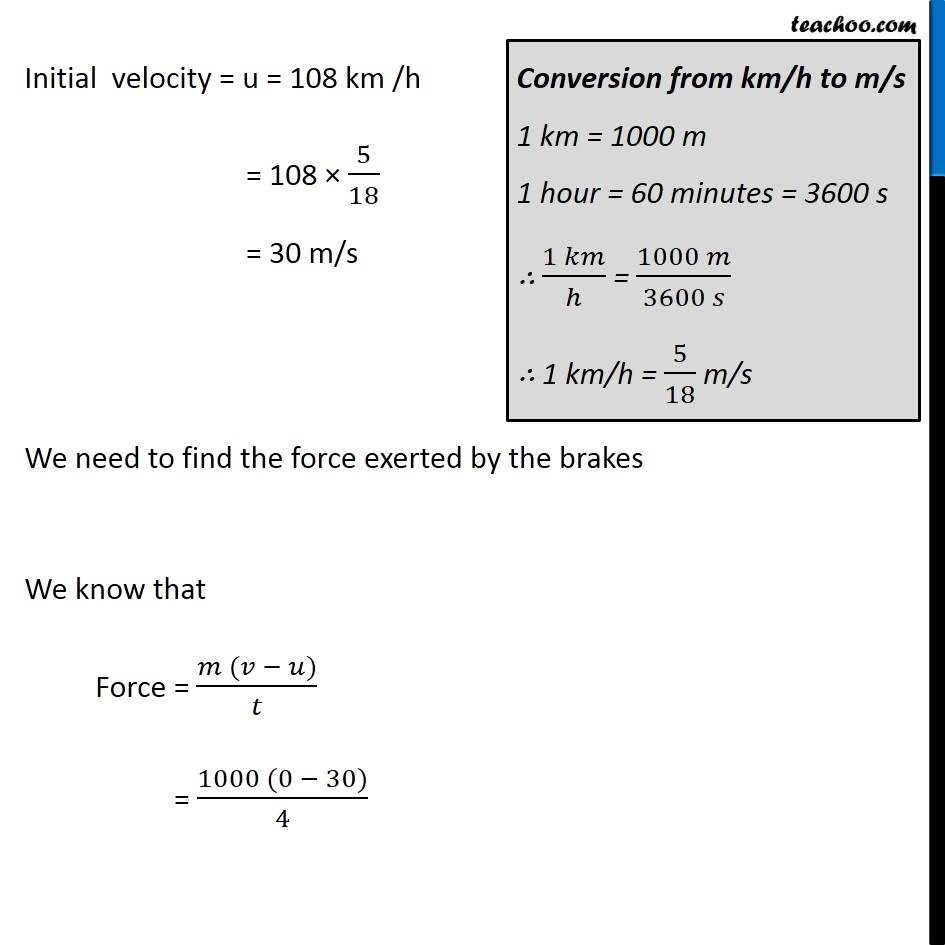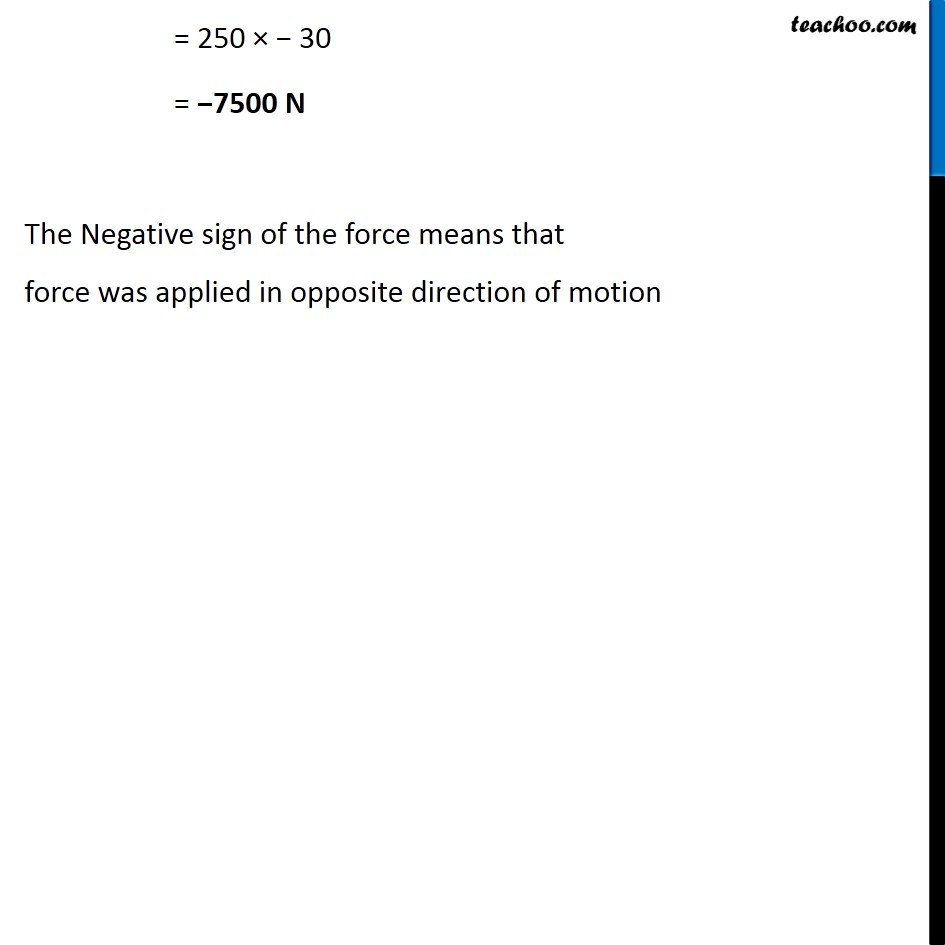Examples from NCERT Book

Class 9
Chapter 9 Class 9 - Force and Laws Of MotionLearn in your speed, with individual attention - Teachoo Maths 1-on-1 Class

### Transcript

Example 9.3 A motorcar is moving with a velocity of 108 km/h and it takes 4 s to stop after the brakes are applied. Calculate the force exerted by the brakes on the motorcar if its mass along with the passengers is 1000 kg. Given Mass of the motor car along with passengers = m = 1000 kg Initial velocity = u = 108 km/h Time taken = 4s Since the car stops, Final velocity = v = 0 m/s Converting initial velocity in m/s Initial velocity = u = 108 km /h = 108 × 5/18 = 30 m/s Conversion from km/h to m/s 1 km = 1000 m 1 hour = 60 minutes = 3600 s ∴ (1 𝑘𝑚)/ℎ = (1000 𝑚)/(3600 𝑠) ∴ 1 km/h = 5/18 m/s We need to find the force exerted by the brakes We know that Force = (𝑚 (𝑣 − 𝑢))/𝑡 = (1000 (0 − 30))/4 = 250 × − 30 = −7500 N The Negative sign of the force means that force was applied in opposite direction of motion